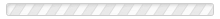Home » EncyclopediaSearch:
(clear)
• o
• open sytem
• operator
In mathematics, “operator” has two main definitions. 1) An operator is a mathematical symbol, for example +, that represents(...) Read More
• orbital
In the early 1900's, when physicists were first probing the insides of the atom, they thought that electrons might travel(...) Read More
• orbital angular momentum
(Symbol: ɭ or L). Electrons have two types of rotational motion: orbital angular momentum and spin. Orbital angular momentum(...) Read More
• ordinary matter
Scientists call the everyday matter of our world, such as tables and chairs, “ordinary matter.” Ordinary matter is made up(...) Read More
• oscillation
Definition (1): An oscillation is a repetitive back-and-forth motion. A pendulum swinging back and forth is an everyday(...) Read More
• oscillator
An oscillator is something that oscillates. Something that vibrates. See oscillation for more information. [caption(...) Read More
• p
• particle
In everyday speech, a particle is a bit of matter. I might say that there is particle of dirt on the lens of my glasses.(...) Read More
• particle-wave duality
When a photon hits your eye, it’s a particle. When it hits your eye, it interacts with a particular electron in the retina(...) Read More
• period
If you were standing on a dock and water waves were coming at you, the period would be the time interval between waves. In(...) Read More
• Periodic Table of the Elements
The Periodic Table of the Elements lists all the elements, over 100 of them, in a specific sequence. This includes the(...) Read More
• physical constant
The constant c, the speed of light in a vacuum, is an example of a physical constant. It is a constant of nature, always(...) Read More
• pi
Pi is symbolized π and pronounced like "pie." It is the mathematical symbol for the number 3.14159…, which goes on(...) Read More
• Pilot Wave Interpretation of quantum mechanics
This interpretation of quantum mechanics is also called the "Bohmian Interpretation." It was originally proposed by(...) Read More
• probability amplitude
“Probability amplitude” is a term used in quantum physics. It’s a number that appears in Schrodinger’s Wave Equation. Let’s(...) Read More
• probability wave
Max Born, one of the early quantum physicists in the 1920's and '30s, proposed that between detections, quantum particles(...) Read More
• q
• QFT
See Quantum Field Theory. Read More
• quantities of dimension one
For “quantities of dimension one,” see “dimensionless number.” Read More
• quantum
One definition of “quantum” is: a tiny packet of energy at the atomic level. A quantum is the smallest possible unit of(...) Read More
• quantum angular momentum
For quantum angular momentum, see angular momentum. Read More
• quantum decoherence
For quantum decoherence, see  decoherence. Read More
• quantum entanglement
In quantum physics, if two particles are entangled, their behavior is correlated. In addition, if a physicist obtains(...) Read More
• Quantum Field Theory
Quantum Field Theory (QFT) is the current theory of how atomic and subatomic particles behave. It is the most up-to-date(...) Read More
• quantum mechanics
In quantum mechanics, physicists study how tiny particles behave. These include atoms, the components of atoms like(...) Read More
• quantum number
Electrons have a few handfuls of properties. Four have been selected as the electron’s “quantum numbers.” The quantum(...) Read More
• quantum physics
For an understanding of quantum physics, see the definition of "quantum mechanics." Often, the two terms are used to mean(...) Read More
• quantum randomness
Many quantum physicists view quantum mechanics as differing from classical physics in a fundamental way—quantum mechanics is(...) Read More
• quantum realm
A number of interpretations of quantum mechanics postulate a “quantum realm.” These include the Transactional(...) Read More
• quantum spin